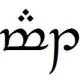# Getting The Ordinal Suffix Of A Number Using ColdFusion

Author: Steven Neiland
Published:

Warning: This blog entry was written two or more years ago. Therefore, it may contain broken links, out-dated or misleading content, or information that is just plain wrong. Please read on with caution.

I would consider this a very simple problem to solve. However after recently coming across a solution for this in the wild that was shall we say less than optimal, I decided that maybe it was worth sharing my solution for any new developers.

## The Complete Function

First here is my solution to the problem. See below for a break down.

`<cffunction name="ordinalSuffix" access="public" output="false" returntype="string">      <cfargument name="theNumber" type="numeric" required="true">            <cfset var suffix = "">            <cfif theNumber GTE 10 AND mid(theNumber,len(theNumber)-1,1) EQ "1">            <cfset suffix = "th">      <cfelseif theNumber GT 0>            <cfswitch expression="#right(theNumber,1)#">                  <cfcase value="1">                        <cfset suffix = "st">                  </cfcase>                  <cfcase value="2">                        <cfset suffix = "nd">                  </cfcase>                  <cfcase value="3">                        <cfset suffix = "rd">                  </cfcase>                  <cfdefaultcase>                        <cfset suffix = "th">                  </cfdefaultcase>            </cfswitch>      </cfif>            <cfreturn suffix></cffunction>`

## Breaking It Down

So what is it doing here. First we check if the number ends with two digits representing values between "10" and "19" by using the string functions mid() and len() to get the second last digit of the number. If true then the suffix is always "th".

`<cfif theNumber GTE 10 AND mid(theNumber,len(theNumber)-1,1) EQ "1">      <cfset suffix = "th">`

For every other number greater than zero we get the last digit of the number using the right() string function.

`<cfelseif theNumber GT 0>      <cfswitch expression="#right(theNumber,1)#">`

Based on the value of that last digit we set the suffix accordingly.

`            <cfcase value="1">                  <cfset suffix = "st">            </cfcase>            <cfcase value="2">                  <cfset suffix = "nd">            </cfcase>            <cfcase value="3">                  <cfset suffix = "rd">            </cfcase>            <cfdefaultcase>                  <cfset suffix = "th">            </cfdefaultcase>      </cfswitch></cfif>`

## Alternative Solution

Here is an alternative solution as suggestion by Matt Gutting. I tested it against my solution and it does give a small performance increase when you get up to several thousand calls. On a loop of 10,000 I got a difference of 300ms (mine) vs 180ms (matts).

`<cffunction name="ordinalSuffix" access="public" output="false" returntype="string">      <cfargument name="theNumber" type="numeric" required="true">            <cfset var suffix = "">            <cfif int(theNumber / 10) mod 10 is 1>            <cfset suffix = "th">      <cfelseif theNumber GT 0>            <cfswitch expression="#theNumber mod 10#">                  <cfcase value="1">                        <cfset suffix = "st">                  </cfcase>                  <cfcase value="2">                        <cfset suffix = "nd">                  </cfcase>                  <cfcase value="3">                        <cfset suffix = "rd">                  </cfcase>                  <cfdefaultcase>                        <cfset suffix = "th">                  </cfdefaultcase>            </cfswitch>      </cfif>            <cfreturn suffix></cffunction>`

# Related Blog PostingsMatt Gutting
Wednesday, April 25, 2012 at 10:29:39 PM Coordinated Universal Time

Very nice. Two things that I would have done differently, but not necessarily better: First, I think this is one of the few times where I would have used CFScript. Maybe. Second, instead of picking apart theNumber as a string of digits, I would have taken advantage of the fact that it's described in cfargument as a numeric. Thus, to see whether it's between 10 and 19, I would have replaced

<cfif theNumber GTE 10 AND mid(theNumber,len(theNumber)-1,1) EQ "1">

with

<cfif int(theNumber / 10) mod 10 is 1>

and

<cfswitch expression="#right(theNumber,1)#">

Just my approach.
with

<cfswitch expression="#theNumber mod 10#">Steven Neiland
Thursday, April 26, 2012 at 12:43:03 AM Coordinated Universal Time

Thanks Matt.

Im not a fan of cfscript in general. I like to keep my code consistent and because I find cfquery clunky in script I am unlikely to switch.

I like your approach to finding the 10-19 range. It does give a small performance boost of around 400ms over a cycle of 10000 permutations. Its small but every bit counts.

The one point in favour of my solution is that it is easy to understand for new developers the logic without having to go into explaining the math.Jules Gravinese
Friday, May 18, 2018 at 11:01:17 PM Coordinated Universal Time

Do you think you'd get a small speed boost by using CFRETURN instead of CFSET? <cfif int(theNumber / 10) mod 10 is 1> <cfreturn "th"> <cfelseif theNumber GT 0> <cfswitch expression="#theNumber mod 10#"> <cfcase value="1"> <cfreturn "st"> </cfcase> <cfcase value="2"> <cfreturn "nd"> </cfcase> <cfcase value="3"> <cfreturn "rd"> </cfcase> <cfdefaultcase> <cfreturn "th"> </cfdefaultcase> </cfswitch> </cfif>Steven Neiland
Saturday, February 2, 2019 at 12:16:53 AM Coordinated Universal Time

Hey Jules, Im sure there is always scope to eek out more performance, but at some point you have to look up and work on the bigger picture. Micro optimizations are all well and good but are they worth it?Scott Bennett
Friday, November 18, 2022 at 8:14:08 PM Coordinated Universal Time

I found this today in my searching, and ended up rolling my own function that uses cfscript and basically does the same thing, but with less code: function OrdinalSuffix(n) { return arrayfind([1,2,3],n%10)&&!arrayfind([11,12,13],n%100)?listgetat('st,nd,rd',n%10):'th'; }